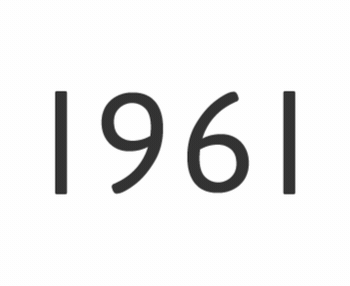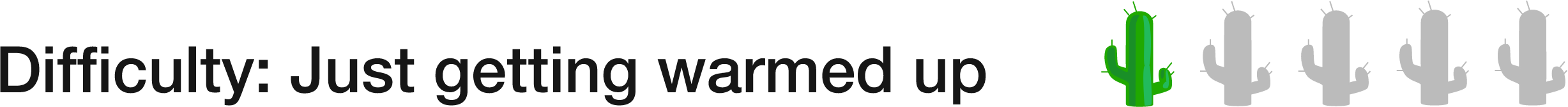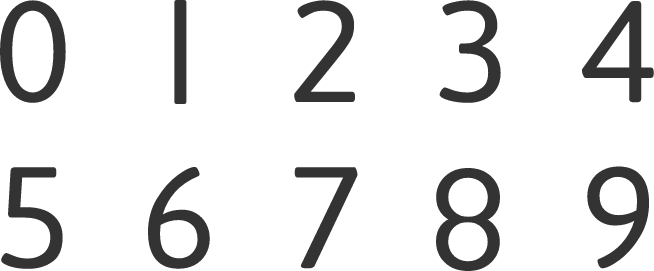# 74 of 100: Number FlipA strobogrammatic number is one where (with certain fonts) the number looks the same when rotated 180 degrees (an example is shown above, the year 1961). The possible digits are 0, 1, 6, 8, and 9.

When is the next year (after 2017) that will be strobogrammatic?While some fonts vary, you can assume 0, 1, 6, 8, and 9 (and only these digits) are all written in a way to allow a strobogrammatic arrangement.×